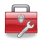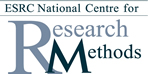# Using Statistical Regression Methods in Education Research# Module 3 - Multiple Linear Regression

### Objectives

1. Understand the strength of Multiple Linear Regression (MLR) in untangling cause and effect relationships
2. Understand how MLR can answer substantive questions within the field of educational research, using the LSYPE dataset for examples
3. Understand the assumptions underlying MLR and how to test they are met
4. Understand how to explore interaction effects between variables
5. Be able to implement and interpret MLR analyses using SPSS
6. Appreciate the applications of MLR in educational research, and possibly in your own research## Start Module 3: Multiple Linear Regression###### Using multiple explanatory variables for more complex regression models.You can jump to specific pages using the contents list below. If you are new to this module start at the overview and work through section by section using the 'Next' and 'Previous' buttons at the top and bottom of each page. Be sure to tackle the exercise and the quiz to get a firm understanding.

This Module is also available as a downloadable PDF for those of you who prefer to read from a page. You do miss out on the glossary and some of the site's more interactive features though... Module 3: Multiple Linear Regression#### Contents

3.1 Overview

3.2 The Multiple Linear Regression Model

3.3 Assumptions of Multiple Linear Regression

3.4 Using SPSS to model the LSYPE data

3.5 A model with a ordinal explanatory variable (Model 1)

3.6 Adding dichotomous nominal explanatory variables (Model 2)

3.7 Adding nominal variables with more than two categories (Model 3)

3.8 Predicting scores using the regression model

3.9 Refining the model: treating ordinal variables as dummy variables (Model 4)

3.10 Comparing coefficients across models

3.11 Exploring interactions between a dummy and a continuous variable (Model 5)

3.12 Exploring interactions between two nominal variables (Model 6)

3.13 A value added model (Model 7)

3.14 Model diagnostics and checking your assumptions

Quiz

Exercise

 HomeModules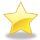Site Guide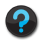Module 3 Contents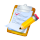Resources